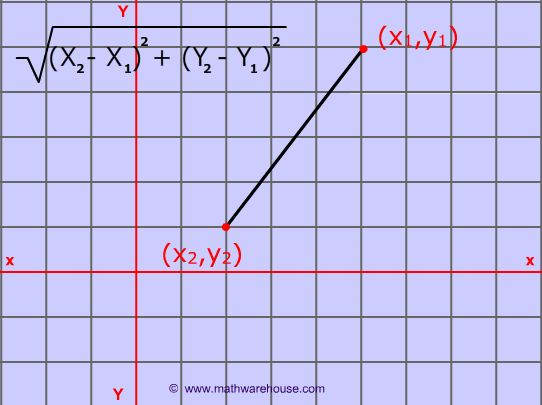# Java Program To Calculate Distance Between Two Points | 3 Ways

Java program to calculate the distance between two points. The code has been written in five different formats using standard values, taking inputs through scanner class, command line arguments, while loop and, do while loop, creating a separate class. If you nay doubts related to the information that we shared do leave a comment here at the end of the post.

How to calculate the distance between two points?

The distance between two points formula derived from the Pythagorean Theorem.

What is the formula to find the distance between two points?

A: Here is the formula to find the distance between two points:

To find the distance between two points (x1,y1) and (x2,y2), all that you need to do is use the coordinates of these ordered pairs and apply the formula pictured below.source

1.Java Program using standard values

There you go formula-based program with sample output.

output:

2. Taking inputs through scanner class

Sample code #2: Taking inputs through scanner class.

output:

3. Using command line arguments

Taking inputs through command line arguments. Do check out the complete guide on command line arguments here.

output:

4. User Define Method

By using a user-defined method.

output:

5. Creating a separate class

Through creating a separate class( ) and taking inputs through scanner class.

output:

x

## X Star Pattern Java Program – Patterns

Java program to print X star pattern program – We have written the below print/draw ...